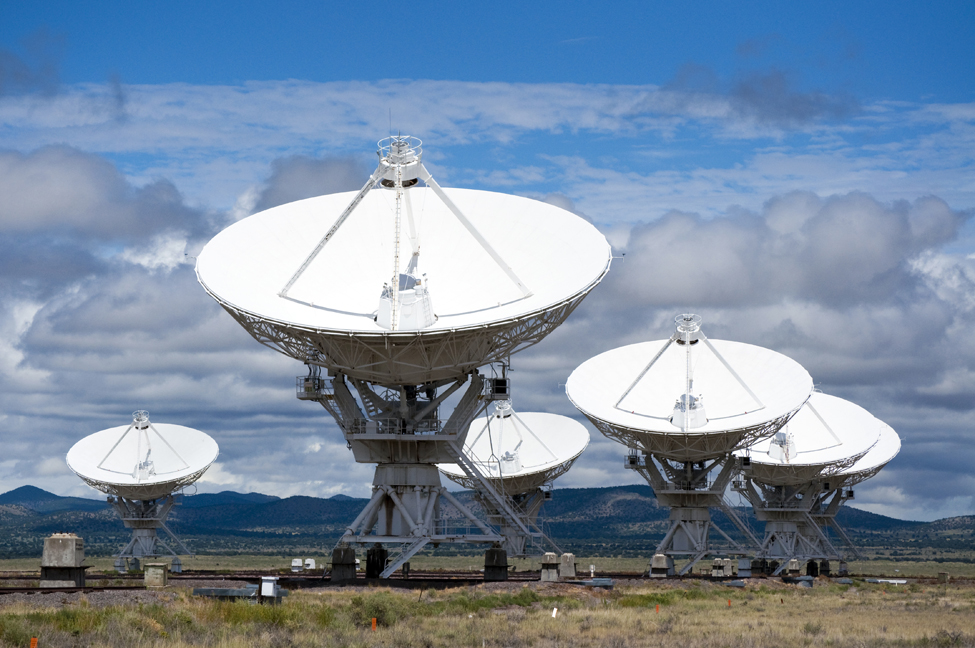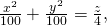Vectors in Space

# 7 Introduction

The Karl G. Jansky Very Large Array, located in Socorro, New Mexico, consists of a large number of radio telescopes that can collect radio waves and collate them as if they were gathering waves over a huge area with no gaps in coverage. (credit: modification of work by CGP Grey, Wikimedia Commons)Modern astronomical observatories often consist of a large number of parabolic reflectors, connected by computers, used to analyze radio waves. Each dish focuses the incoming parallel beams of radio waves to a precise focal point, where they can be synchronized by computer. If the surface of one of the parabolic reflectors is described by the equationwhere is the focal point of the reflector? (See (Figure).)

We are now about to begin a new part of the calculus course, when we study functions of two or three independent variables in multidimensional space. Many of the computations are similar to those in the study of single-variable functions, but there are also a lot of differences. In this first chapter, we examine coordinate systems for working in three-dimensional space, along with vectors, which are a key mathematical tool for dealing with quantities in more than one dimension. Let’s start here with the basic ideas and work our way up to the more general and powerful tools of mathematics in later chapters.